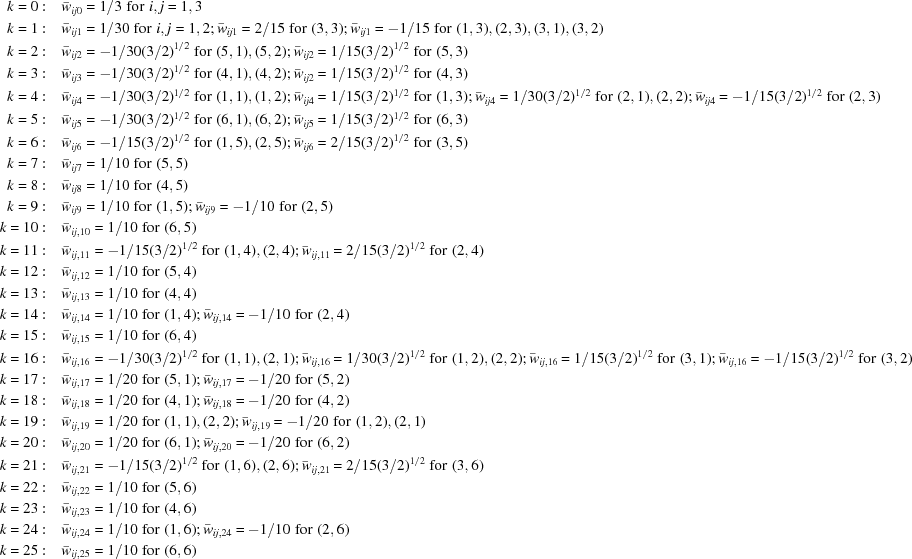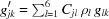JOURNAL OFAPPLIEDCRYSTALLOGRAPHY
ISSN: 1600-5767

## Elastic strain and stress determination by Rietveld refinement: generalized treatment for textured polycrystals for all Laue classes. Second corrigendum

aNational Institute for Materials Physics, PO Box MG 7, Bucharest, Romania, and bDepartment of Physics and Astronomy, University of Denver, 2112 East Wesley Avenue, Denver, CO 80208, USA
*Correspondence e-mail: balzar@du.edu

(Received 7 October 2014; accepted 7 October 2014; online 4 November 2014)

This corrigendum (C2) replaces the first corrigendum (C1; Popa & Balzar, 2012), which was published to correct errors in the article by Popa & Balzar (2001). The basic equation considered in C1 is the integral of spherical harmonics over the crystallites contributing to the Bragg reflection. In C1, this integral is represented by equation (1), which is the expression (14.160) originally calculated by Bunge (1982). In C1, we added the factor ( - 1)n to that expression. This factor resulted from a coding error that, unfortunately, we discovered only recently in the computer routine used to calculate such integrals. With the correct coding, the factor ( - 1)n disappears, and the Bunge (1982) equation (14.120) is correct.

The necessary corrections in the paper by Popa & Balzar (2001) are the following:

(1) Table 1: multiply the right side of each equation by 2.

(2) Table 15: delete the factor 2 in the right side of the equations for R0 and R1.

(3) Several errors in Table 16. The correct table is shown as Table 1of the present article.

 Table 1The corrected version of Table 16(4) The first unnumbered equation after equation (24) becomes.

### ReferencesJOURNAL OFAPPLIEDCRYSTALLOGRAPHY